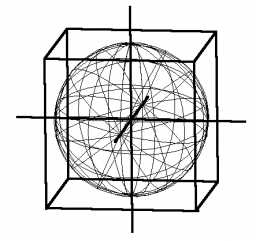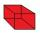# Calculate 3

Calculate the cube volume whose edge is 3x-1,3x-1,3x-1

Result

V = (Correct answer is: 27 x^3 - 27 x^2 + 9 x - 1)### Step-by-step explanation:Did you find an error or inaccuracy? Feel free to write us. Thank you!Tips to related online calculators
Tip: Our volume units converter will help you with the conversion of volume units.

## Related math problems and questions:

• Cube surfce2volumeCalculate the volume of the cube if its surface is 150 cm2.
• The cubeThe cube has a surface area of 216 dm2. Calculate: a) the content of one wall, b) edge length, c) cube volume.
• Area of a cubeCalculate the surface area of a cube if its volume is equal to 729 cubic meters.
• Volume and areaWhat is the volume of a cube which has an area of 361 cm2?
• Cube V2SThe volume of the cube is 27 dm cubic. Calculate the surface of the cube.
• Regular square prismThe volume of a regular square prism is 192 cm3. The size of its base edge and the body height is 1: 3. Calculate the surface of the prism.
• Length of the edgeFind the length of the edge of a cube with a cm2 surface and a volume in cm3 expressed by the same number.
• TerezaThe cube has an area of base 256 mm2. Calculate the edge length, volume, and area of its surface.
• The cubeThe cube has a surface area of 486 m ^ 2. Calculate its volume.
• Cube edgesIf the edge length of the cube increases by 50%, how does the volume of this cube increase?
• Cube surface and volumeFind the surface of the cube with a volume of 27 dm3.
• Equilateral cylinderEquilateral cylinder (height = base diameter; h = 2r) has a volume of V = 199 cm3 . Calculate the surface area of the cylinder.
• Cube basicsHow long is the edge length of a cube with volume 15 m3?
• Cylinder - areaThe diameter of the cylinder is one-third the length of the height of the cylinder. Calculate the surface of cylinder if its volume is 2 m3.
• Special cubeCalculate the edge of cube, if its surface and its volume is numerically equal number.
• Body diagonalThe cuboid has a volume of 32 cm3. Its side surface area is double as one of the square bases. What is the length of the body diagonal?
• Cube 1-2-3Calculate the volume and surface area of the cube ABCDEFGH if: a) /AB/ = 4 cm b) perimeter of wall ABCD is 22 cm c) the sum of the lengths of all edges of the cube is 30 cm.